# Atiyah-Floer conjecture

A conjecture relating the instanton Floer homology of suitable three-dimensional manifolds with the symplectic Floer homology of moduli spaces of flat connections over surfaces, and hence with the quantum cohomology of such moduli spaces. It was originally stated by M.F. Atiyah for homology-spheres in [a1]. The extension of the conjecture to the case of mapping cylinders was prompted by A. Floer and solved in this case by S. Dostoglou and D. Salamon in [a3].

Instanton Floer homology for three-dimensional manifolds was introduced by Floer in [a10]. Let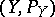be a pair consisting of a closed oriented-dimensional manifoldand an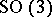-bundle. If eitheris a homology-sphere or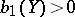and the second Stiefel–Whitney class, then the instanton Floer homologyis defined as the homology of the Morse-type complex constructed out of the Chern–Simons functional. The critical points are flat connections and the connecting orbits are anti-self-dual connections on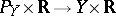decaying exponentially to flat connections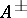when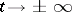.

The symplectic Floer homology for Lagrangian intersections was introduced by Floer in [a11]. Let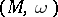be a symplectic manifold which is monotone and simply connected. Let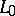and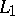be Lagrangian submanifolds of. Then there are Floer homology groups. Now the critical points are the intersection points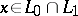and the connecting orbits are-holomorphic strips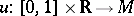with,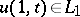and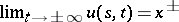, where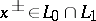and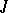is an almost-complex structure compatible with the symplectic form.

Letbe a closed oriented surface of genusand let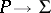be the trivial-bundle. Then the moduli spaceof flat connections onis symplectic and smooth except at the trivial connection. Now, let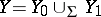be a Heegaard splitting of a homology-sphere and consider the trivial-bundle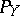on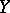. Then the flat connections on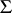which extend to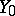define a Lagrangian subspace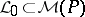, and analogously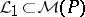. Taking care of the singularity one may define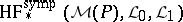. The Atiyah–Floer conjecture reads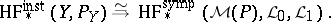(a1)

This was originally conjectured by Atiyah in [a1]. An overview of the problem appears in [a8]. The problem is still open (as of 2000).

The symplectic Floer homology for a symplectic mapping was introduced by Floer in [a12]. Let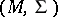be a symplectic manifold which is monotone and simply connected. Letbe a symplectomorphism. Then the symplectic Floer homology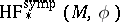can be defined as the Morse-type theory where the critical points are the fixed points ofand the connecting orbits are-holomorphic stripswith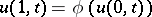which converge to fixed points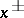ofas. For, Floer proved [a12] that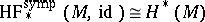. Moreover, there is a natural ring structure for the symplectic Floer homology [a8], and in [a7] it is proved that there is an isomorphism of rings, where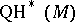is the quantum cohomology of.

Letbe a closed oriented surface of genus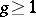and let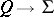be the non-trivial-bundle. The moduli space of flat connectionsis a smooth symplectic manifold. Consider the mapping cylinderof a diffeomorphism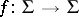. This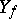fibres over the circle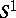with fibre. Liftto a bundle mapping. This gives an-bundle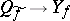. On the other hand,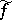induces a mapping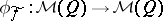. The Atiyah–Floer conjecture for mapping cylinders was proposed by Floer [a4] and reads: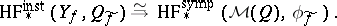(a2)

In [a3], Dostoglou and Salamon prove the existence of an isomorphism between these two Floer homologies by constructing an isomorphism at the chain level and identifying the boundary operators. The idea is named adiabatic limit and consists of stretchingin the direction orthogonal to.

A very important case is that of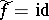. Then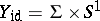and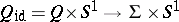is the-bundle with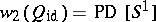. Therefore,(a3)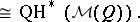Both Floer homologies have natural product structures, introduced by S.K. Donaldson (see [a8]). A stronger version of the Atiyah–Floer conjecture establishes that (a3) is an isomorphism of rings.

The existence of such an isomorphism has been proved by V. Muñoz in [a5], [a6] by giving an explicit presentation of both rings in terms of the natural generators of the cohomology ofand using the relationship of instanton Floer homology of-manifolds with Donaldson invariants of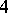-manifolds [a2]. Also, in [a9] Salamon proves that the adiabatic limit isomorphism is indeed a ring isomorphism.